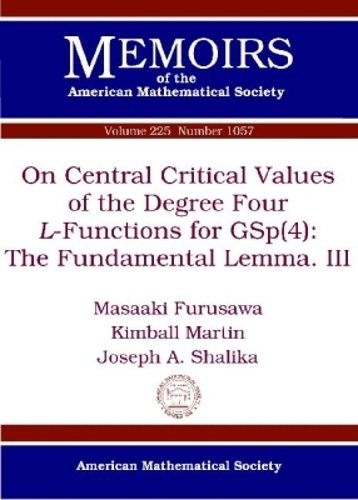•# On Central Critical Values of the Degree Four L-Functions for GSp(4): The Fundamental Lemma. III - Memoirs of the American Mathematical Society (Paperback)

(author), (author)
£77.95
Paperback 134 Pages / Published: 30/09/2013
• We can order this

Usually dispatched within 2 weeks

Some time ago, the first and third authors proposed two relative trace formulas to prove generalisations of Bocherer's conjecture on the central critical values of the degree four $L$-functions for $\mathrm{GSp}(4)$, and proved the relevant fundamental lemmas. Recently, the first and second authors proposed an alternative third relative trace formula to approach the same problem and proved the relevant fundamental lemma. In this paper the authors extend the latter fundamental lemma and the first of the former fundamental lemmas to the full Hecke algebra. The fundamental lemma is an equality of two local relative orbital integrals. In order to show that they are equal, the authors compute them explicitly for certain bases of the Hecke algebra and deduce the matching.

Publisher: American Mathematical Society
ISBN: 9780821887424
Number of pages: 134